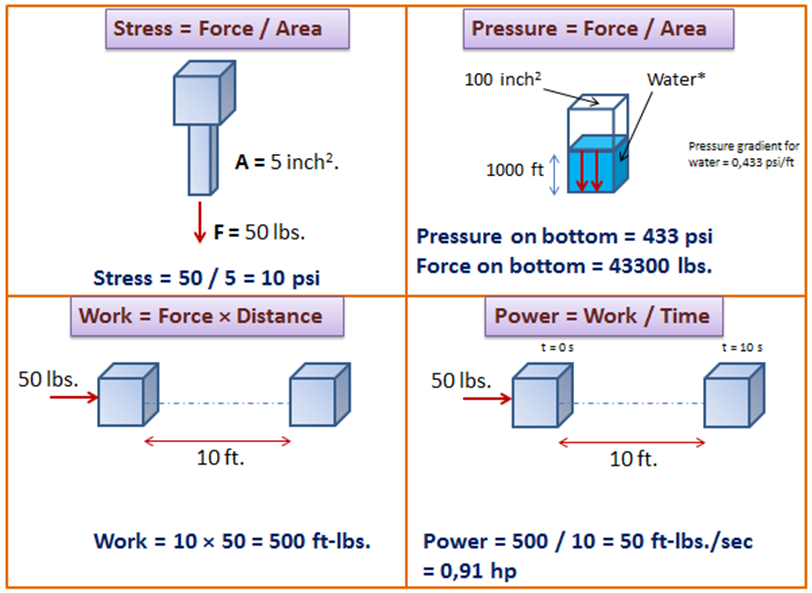# Fundamental principles of physics used in artificial lift design

In the present article, we discuss a couple of physical quantities used in artificial lift design such as the concept of stress, pressure, work, power, energy, machine, and efficiency.Stress is defined as force divided by area. So that, to reduce the stress you can either reduce the force or increase the cross sectional area it acts on.

### Stress = Force / Area

Pressure is also defined as force divided by area but is primarily used to understand the effect of fluids on surfaces.

### Pressure = Force / Area

If we have a bottle filled with 1 foot of water, the pressure at the bottom of the bottle will be 1 psi.

Work is defined as what a force accomplishes when it acts on a body for a certain distance.

### Work = Force × Distance

For example, if you need to push a box 10 feet by applying a force of 50 lbs. how much work have you done?  The answer is 500 ft-lbs. of work (10 ft ×50 lbs.).

Notice that the definition of work does not include time.

Power is defined as work divided time.

### Power = Work / Time

Let’s take the previous example; if we measure the time it takes to move the box and we found it takes 10 seconds. Therefore, the power required is 500 (ft-lbs)/sec or 0.91 horsepower (1 hp = 550 (ft-lbs)/s = 745.7 watts).

The faster the box is moving, the more power is required.

Energy is defined as the capability of a physical system to perform work. It exists in several forms such as heat, kinetic energy, potential energy, mechanical energy, electrical energy, heat, etc.

Machine: A machine is a device or system that can convert energy (electrical energy for example) into work (lifting reservoir fluid to the surface for example).

Efficiency is defined as the ratio of the useful work done divided by the energy used.

In a rod pumping system, the rod pumping system itself is a machine, which increases the potential energy of the produced fluid. The useful work is the work required to bring the produced fluid to the surface. The energy used is the energy consumed by the rod pumping system while doing the useful work.